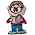Wednesday, September 23, 2009

How Quickly Can You Do It?

Which is larger: one-third times one-third of a dozen dozen, or one-third dozen halved and cubed?

1.Math in public...

1/3 * 1/3 * (12*12)
12 * 12 = 144
1/3 * 144 = 48
1/3 * 48 = 16

[(1/3 * 12) * 1/2]^3
1/3 * 12 * 1/2 = 2
2^3 = 2 * 2 * 2 = 8

So I'd guess one-third times one-third of a dozen dozen

is larger

than one-third dozen halved and cubed.

2.Slightly different method, same answer: the first one is larger. If you wanted to do it really quickly, the first one is squared before dividing, but the second one is cubed after dividing. I think the time of division is the key.

3.(1/3)*(1/3)(12*12)=16
(1/3)(12)(.5)=8
So the first one would be larger

4.Wait, the second one should've looked like (1/3)(12)(.5)^3=8

5.A mathematician might have generalized and come to the wrong conclusion:
If we let X = 12/3, then the two formulas are
X^2 and (X/2)^3. For large enough X, the cubed is going to get much larger. But for X small enough, the first is going to be bigger. In this case, X = 4 is small enough for the first to be bigger. As an aside, at X = 8 the two functions are equal.

Enough lecturing... USAF Pilot, Charil and satyrnxoxo had it right. 16 is bigger than 8.

Leave your answer or, if you want to post a question of your own, send me an e-mail. Look in the about section to find my e-mail address. If it's new, I'll post it soon.

Please don't leave spam or 'Awesome blog, come visit mine' messages. I'll delete them soon after.

Enter your Email and join hundreds of others who get their Question of the Day sent right to their mailbox

The Lamplight Manor Puzz 3-D